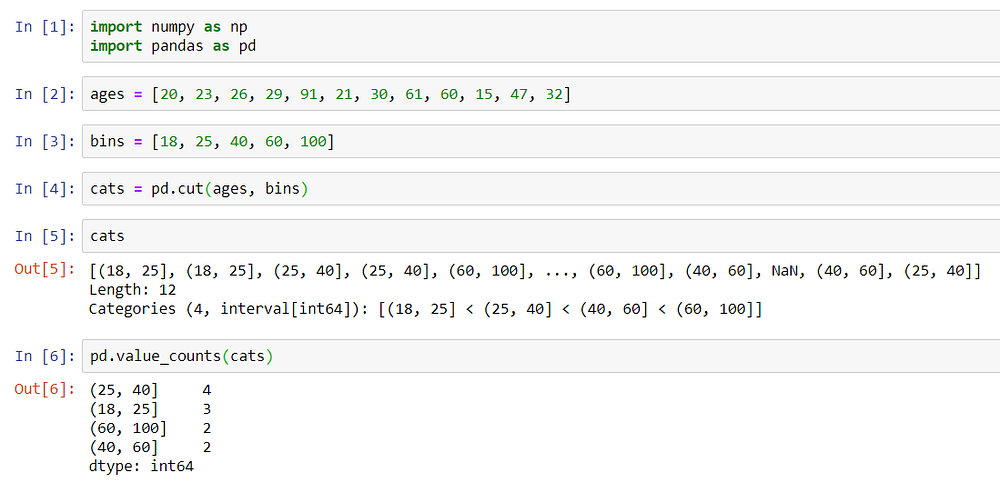### What is defined as a noise in data?

Suppose that we have a dataset in which we have some measured attributes. Now, these attributes might carry some random error or variance. Such errors in attribute values are called as noise in the data.

If such errors persist in our data, it will return inaccurate results.

### The Binning Method

In this method, the set of data values are sorted in an order, grouped into “buckets” or “bins” and then each value in a particular bin is smoothed using its neighbor, i.e. its surrounding values.

It is also said that the binning method does local smoothing because it consults its nearby (neighbors) values to smooth the values of the attribute.

Let us take an example -

Suppose that we have a set of following values: [4, 8, 15, 21, 21, 24, 25, 28, 34]

We will divide this dataset into sets of equal frequency.

Bin1: 4, 8, 15

Bin2: 21, 21, 24

Bin3: 25, 28, 34

There are several ways of binning the values -

#### Smoothing by bin means

In this method, all the values of a particular bin are replaced by the mean of the values of that particular bin.

Mean of 4, 8, 15 = 9

Mean of 21, 21, 24 = 22

Mean of 25, 28, 34 = 29

Bin1: 9, 9, 9

Bin2: 22, 22, 22

Bin3: 29, 29, 29

#### Smoothing by bin medians

In this method, all the values of a particular bin are replaced by the median of the values of that particular bin.

Median of 4, 8, 15 = 8

Median of 21, 21, 24 = 21

Median of 25, 28, 34 = 28

Bin1: 8, 8, 8

Bin2: 21, 21, 21

Bin3: 28, 28, 28

#### Smoothing by bin boundaries

In this method, all the values of a particular bin are replaced by the closest boundary of the values of that particular bin.

Therefore using this technique results in the following bins.

Bin1: 4, 4, 15

Bin2: 21, 21, 24

Bin3: 25, 25, 34

### Binning a set of values using Pandas